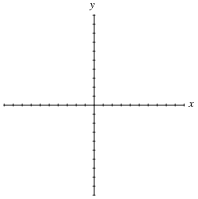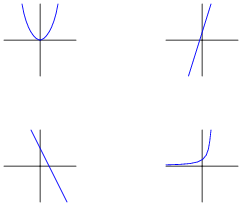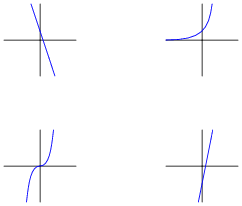# Basic Algebra and Graphing for Electric Circuits

## Mathematics for Electronics

• #### Question 1

Many different equations used in the analysis of electric circuits may be graphed. Take for instance Ohm’s Law for a 1 kΩ resistor:Plot this graph, following Ohm’s Law. Then, plot another graph representing the voltage/current relationship of a 2 kΩ resistor.

• #### Question 2

Many different equations used in the analysis of electric circuits may be graphed. Take for instance Ohm’s Law for a variable resistor connected to a 12 volt source:Plot this graph, following Ohm’s Law.

• #### Question 3

Observe the following equivalence:

 43 ×42 = (4 ×4 ×4) ×(4 ×4)

Since all operations are the same (multiplication) and reversible, the parentheses are not needed. Therefore, we may write the expression like this:

 4 ×4 ×4 ×4 ×4

Of course, the simplest way to write this is 45, since there are five 4’s multiplied together.

Expand each of these expressions so that there are no exponents either:

35 ×32 =
104 ×103 =
82 ×83 =
201 ×202 =

After expanding each of these expressions, re-write each one in simplest form: one number to a power, just like the final form of the example given (45). From these examples, what pattern do you see with exponents of products. In other words, what is the general solution to the following expression?

 am ×an =

• #### Question 4

Observe the following equivalence:

 43 42 = 4 ×4 ×4 4 ×4

It should be readily apparent that we may cancel out two quantities from both top and bottom of the fraction, so in the end we are left with this:

 4 1

Re-writing this using exponents, we get 41.

Expand each of these expressions so that there are no exponents either:

$$(\frac{3^5}{3^2})$$ =

$$(\frac{10^6}{10^4})$$ =

$$(\frac{8^7}{8^3})$$ =

$$(\frac{20^5}{20^4})$$ =

After expanding each of these expressions, re-write each one in simplest form: one number to a power, just like the final form of the example given (41). From these examples, what pattern do you see with exponents of products. In other words, what is the general solution to the following expression?

 am an =

• #### Question 5

Observe the following equivalence:

 42 43 = 4 ×4 4 ×4 ×4

It should be readily apparent that we may cancel out two quantities from both top and bottom of the fraction, so in the end we are left with this:

 1 4

Following the rule of $$(\frac{a^m}{a^n} = a^{m-n})$$, the reduction of $$(\frac{4^2}{4^3})$$ should be 4−1. Many students find this confusing, as the intuitive concept of exponents (how many times a number is to be multiplied by itself) fails here. How in the world do we multiply 4 by itself -1 times?!

Expand each of these expressions so that there are no exponents either:

$$(\frac{3^2}{3^5})$$ =

$$(\frac{10^4}{10^6})$$ =

$$(\frac{8^3}{8^7})$$ =

$$(\frac{20^4}{20^5})$$ =

After expanding each of these expressions, re-write each one in simplest form: one number to a power, just like the final form of the example given (4−1), following the rule $$(\frac{a^m}{a^n} = a^{m-n})$$. From these examples, what easy-to-understand definition can you think of to describe negative exponents?

Also, expand the following expression so there are no exponents, then re-write the result in exponent form following the rule $$(\frac{a^m}{a^n} = a^{m-n})$$:

 53 53

What does this tell you about exponents of zero?

• #### Question 6

When evaluating (calculating) a mathematical expression, what order should you do the various expressions in? In other words, which comes first: multiplication, division, addition, subtraction, powers, roots, parentheses, etc.; and then what comes after that, and after that?

• #### Question 7

Follow proper order of operations to evaluate these expressions:

$$\frac{13 + 2}{3} + 8 =$$

$$25 + (3+2)^2 x 2 =$$

• #### Question 8

Follow proper order of operations to evaluate these expressions:

$$\frac{15 - 3}{3} + 7 =$$

$$20 + (1+3)^2 x 3 =$$

• #### Question 9

When evaluating an expression such as this, it is very important to follow proper order of operations. Otherwise, the correct result will be impossible to arrive at:

 3 log25 + 14

To show what the proper order of operations is for this expression, I show it being evaluated step by step here

 3 log25 + 14

 3 log32 + 14

 3 ×1.5051 + 14

 4.5154 14

 18.5154

Do the same for each of the following expressions:

10 − 25 × 2 + 5
− 8 + 103 × 51
124 × (3 + 11)
21(7 − 4) ×40
$$log \sqrt{6 + 35^2}$$
$$\sqrt{(\frac{220}{16}-2.75)} x 2$$

### Footnotes:

By the way, this is a highly recommended practice for those struggling with mathematical principles: document each and every step by re-writing the expression. Although it takes more paper and more effort, it will save you from needless error and frustration
!

• #### Question 10

Perform the following calculations:

 812 810 = 53 54 = ( 24 ) ( 2−1 ) =

• #### Question 11

Perform the following calculations:

 106 103 = 32 33 = ( 26 ) ( 2−4 ) =

• #### Question 12

The equation for calculating total resistance in a parallel circuit (for any number of parallel resistances) is sometimes written like this:

 Rtotal = (R1−1 + R2−1 + …Rn−1)−1

Re-write this equation in such a way that it no longer contains any exponents.

• #### Question 13

A function is a mathematical relationship with an input (usually x) and an output (usually y). Here is an example of a simple function:

 y = 2x +1

One way to show the pattern of any given function is with a table of numbers. Complete this table for the given values of x:

 x 2x 1 0 1 2 3 4 5

A more common (and intuitive) way to show the pattern of any given function is with a graph. Complete this graph for the same function y = 2x 1. Consider each division on the axes to be 1 unit:• #### Question 14

A famous illustrative story for understanding exponents goes something like this:

A pauper saves the life of a king. In return, the king offers the pauper anything he desires as a reward. The pauper, being a shrewd man, tells the king he does not want much, only a grain of rice today, then double that (two grains of rice) the next day, then double that (four grains of rice) the next day, and so on. The king asks how long he is to give the pauper rice, and the pauper responds by saying one day for every square on a chess board (64 days). This does not sound like much to the king, who never took a math course, and so he agrees.

In just a short amount of time, though, the king finds himself bankrupted to the pauper because the quantity of rice is so enormously large. Such is the nature of exponential functions: they grow incredibly large with modest gains in x.

Graph the pauper’s rice function (y = 2x), with each division on the horizontal axis representing 1 unit and each division on the vertical axis representing 100 units.• #### Question 15

Match each written function (y = …) with the sketched graph it fits best:

 y = 3x + 2 y = 5 − 2x

 y = x2 y = 2x• #### Question 16

Match each written function (y = …) with the sketched graph it fits best:

 y = 5x − 2 y = 1 − 3x

 y = x3 y = 3x### Related Content

Published under the terms and conditions of the Creative Commons Attribution License

1 Comment• B
bjm999 July 15, 2020

Question 3, answer - the ‘+’ symbol is missing from ‘= a^(m+n)’.
Question 7, question and answer - second equation should have a multiplication symbol, not the ‘variable x’.
Question 8, question and answer - second equation should have a multiplication symbol, not the ‘variable x’.
Question 9, question - the ‘+’ symbol is missing between ‘4.5154 ‘+’ 14’.